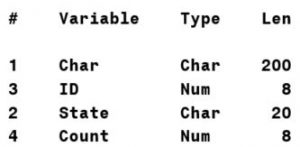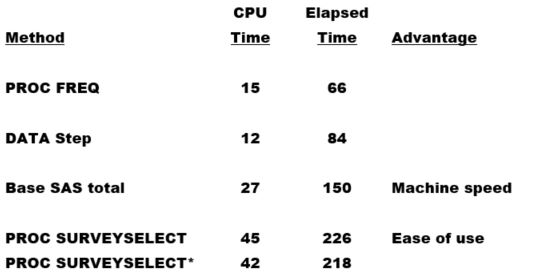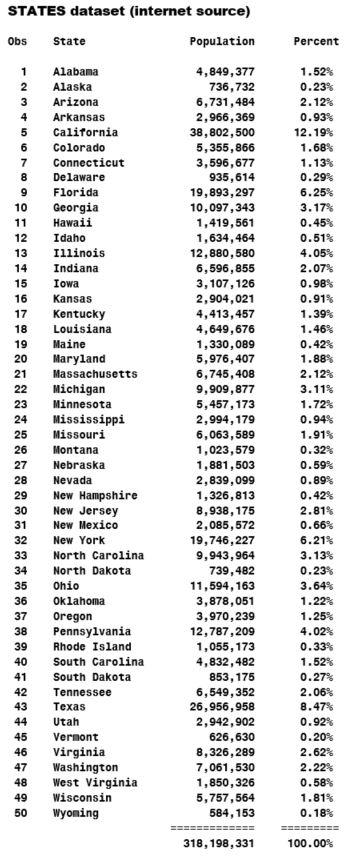In a previous blog, Random Sampling: What's Efficient?, I discussed the efficiency of various techniques for selecting a simple random sample from a large SAS dataset.  PROC SURVEYSELECT easily does the job:

```proc surveyselect data=large out=sample method=srs /* simple random sample */ rate=.01; /* 1% sample rate */ run;```

Note: If you have not read the above-linked blog, I recommend you do so before you continue reading this one.

Now suppose your data includes a STATE variable, and you want to guarantee that your random sample includes the correct proportion of observations from each U.S. state.  You need a stratified random sample.  Again, PROC SURVEYSELECT easily does the job:

```proc surveyselect data=large out=sample1 method=srs /* simple random sample */ rate=.01; /* 1% sample rate */ strata state /* sorted data required */ / alloc=proportional; run;```

When the STRATA statement is used, PROC SURVEYSELECT requires the input dataset to be sorted by the strata column (STATE).

If you have a SAS/STAT license, you can enjoy the ease of use and flexibility of PROC SURVEYSELECT.  If not, or if you're an efficiency hound like me, and you don't mind writing a little code (also like me) you can select a stratified random sample with a super-efficient 2-step BASE SAS program.

Here’s how:

Step 1 of 2:

The PROC FREQ step below reads the LARGE dataset and creates a tiny output dataset, named COUNTS, with one column, named COUNT, and 50 rows, one per US state, sorted by STATE.

```proc freq data=large (keep=state); table state / noprint out=Counts (keep=Count); run;```

The LARGE dataset has 100 million rows and the four columns below:The STATE variable is distributed in approximately the same proportions as the actual population of each US state.  Additionally, the LARGE dataset is sorted by STATE.

Refer to the end of this blog to see how I created the LARGE dataset.

Step 2 of 2:

The DATA step below selects a stratified random sample of exactly 1 million rows (1%) from the LARGE dataset, reading only the selected rows, bypassing the other 99 million rows (99%) for extremely fast performance.  As with PROC SURVEYSELECT, the LARGE dataset must be sorted by the strata column (STATE).  The results are comparable to PROC SURVEYSELECT results.

```data sample2;   drop Need Pool Ratio Row Jump;   set Counts; * One row per strata (US state); Need=round(Count*.01); * 1% sample from each strata; Pool=Count; * Available observations per strata; Jump+Count; * Cumulative count per strata;   do until (Need=0); * Continue until no additional rows needed;   Row+1; * Row number. Every row is considered;   Ratio=Need/Pool; * 1 percent, continuously adjusted;   if rand('uniform') < ratio then do; * Random selection; set large point=Row; * Jump to and read selected row; output; * Output selected row; Need=Need-1; * Subtract 1 from needed rows; end;   Pool=Pool-1; * Subtract 1 from pool of remaining rows;   end;   Row=Jump; * JUMP (created above) to the last ROW of the strata;   run;```

### Efficiency comparison

Times below represent averages, rounded to the nearest second:*I ran PROC SURVEYSELECT a second time, adding the DROP= option below to the SAMPLE1 output dataset, to drop variables otherwise created automatically by PROC SURVEYSELECT:

```(drop=AllocProportion SampleSize ActualProportion SelectionProb SamplingWeight)```

The DATA step below creates the LARGE dataset from the STATES dataset.

```data large;   keep ID State Count Char; length Char \$ 200; set states(keep=state percent);   do ID=1 to 100000000*percent until(count=100000000); * 100M; Count+1; output; end;   run;```

The DATA step below creates the STATES dataset.

```data states;   input @8 State & : \$20. Population : comma13. Percent : percent8.;   format Population comma13. Percent percent9.2;   datalines; Copy and paste the below data here. ;```SharePrincipal Technical Training Consultant

Jim Simon is a principal instructor and course developer for SAS Education. Jim has a bachelor's degree from UCLA and a master's degree from California State University at Northridge. Prior to joining the SAS Irvine office in 1988, Jim was an instructor at Ventura College and a SAS programmer at The Medstat Group in Santa Barbara. Jim's areas of specialization include the DATA step, application development, web enablement, and the SAS macro language. A native of Southern California, Jim enjoys anything in the warm California sun. On weekends, Jim loves jumping in his Corvette, turning up the stereo, and cruising Pacific Coast Highway, top down, South to Laguna Beach or North to his old home town, Santa Barbara.

1.Great macro!
Thanks, Brian!
-Jim

2.Nice, Jim! Here's a macro that does something similar. It's not as fast as your code but the data doesn't have to be sorted.

%macro samplestrat(datain, dataout, samplesize, stratum=);
*Get counts of each value;
proc freq data=&datain noprint;
table &stratum. / out=samplefout;
run;

*For each category, get the value, size you want the sample to be, and the population size for that category;
data _null_;
set samplefout end=eof;
call symputx(cats("condition",strip(put(_n_,8.))),&stratum.);
if index("&samplesize.","%") then call symputx(cats("samplesize",put(_n_,8.)),strip(put(round(%scan(&samplesize.,1,%)/100*count),12.)));
else call symputx(cats("samplesize",put(_n_,8.)),put(%scan(&samplesize.,1,%),12.));
call symputx(cats("nobs",put(_n_,8.)),strip(put(count,12.)));
if eof then call symputx('countlevels',put(_n_,8.));
run;

*SRS within stratum similar to how if there is no stratum;
data &dataout.(drop=samplek1--samplen&countlevels.);
retain %do i=1 %to &countlevels.; samplek&i. &&samplesize&i.. samplen&i. &&nobs&i.. %end; ;
set &datain;
if 0 then ;
%do i=1 %to &countlevels.;
else if &stratum.="&&condition&i.." then do;
if ranuni(0) <= samplek&i./samplen&i. then do;
output;
samplek&i.=samplek&i.-1;
end;
samplen&i.=samplen&i.-1;
end;
%end;
run;
%mend;
%samplestrat(large, sample3, 1%, stratum=state)